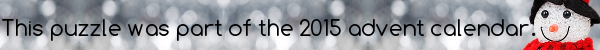mscroggs.co.uk
mscroggs.co.uksubscribe

# Advent calendar 2015

## 4 DecemberPut the digits 1 to 9 (using each digit exactly once) in the boxes so that the sums reading across and down are correct. The sums should be read left to right and top to bottom ignoring the usual order of operations. For example, 4+3×2 is 14, not 10.
 - + = -4 + + + - ÷ = -1 - ÷ × - × = -30 =0 =2 =54
The answer is the product of the digits in the red boxes.
Tags: numbers, grids

## Archive

Show me a random puzzle
▼ show ▼
© Matthew Scroggs 2019